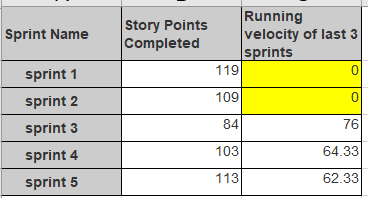# Set default value as '0' for running velocity formula in 1st 2 sprints

Hi,

I want to calculate the running velocity (RV) for the latest 3 sprints, however I want the first 2 RV values defaulted to ‘0’ and calculation should start only from sprint 3 ie:

CASE
WHEN
– for closed sprints only
[Sprint].CurrentMember.getBoolean(‘Closed’) AND
NOT IsEmpty([Sprint].CurrentMember.get(‘Complete date’)) AND
[Measures].[Sprint Story Points at closing] > 0
THEN
AVG(
Tail(
– filter last 3 closed sprints starting from current sprint
Filter(
Cache(ChildrenSet([Sprint].[Agg Sprints])),
Rank([Sprint].CurrentMember,
Cache(ChildrenSet([Sprint].[Agg Sprints]))
)
),
– only sprints with committed story points are retrieved
[Measures].[Sprint Story Points at closing] > 0
), 3
),
[Measures].[Sprint Story Points completed]
)
END

Please let me know how the 1st 2 RV values can be defaulted to zero whereas the other sprints can use this formula mentioned above.Hi @Gomez

Welcome to eazyBI community.

Try this formula to limit the calculation and start calculation velocity from the 3rd sprint.

``````CASE
WHEN
--for closed sprints only
[Sprint].CurrentMember.getBoolean('Closed')
AND
NOT IsEmpty([Sprint].CurrentMember.get('Complete date'))
AND
[Measures].[Sprint Story Points at closing] > 0
AND
Rank([Sprint].CurrentMember,
Cache(ChildrenSet([Sprint].[Agg sprints]))
)>2
THEN
AVG(
Tail(
-- filter last 3 closed sprints starting from current sprint
Filter(
Cache(ChildrenSet([Sprint].[Agg sprints])),
Rank([Sprint].CurrentMember,
Cache(ChildrenSet([Sprint].[Agg sprints]))
)
),
-- only sprints with committed story points are retrieved
[Measures].[Sprint Story Points at closing] > 0
), 3
),
[Measures].[Sprint Story Points completed]
)
ELSE
0
END
``````

It expects that you have a calculated member “Agg sprints” in “Sprint” dimension

Martins / eazyBI support